Next: Transients Up: One-Dimensional Motion Previous: Resonance

Periodic Driving Forces

In the last section, we investigated the response of a one-dimensional dynamical system, close to a stable equilibrium point, to an external force which varies as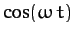. Let us now examine the response of the same system to a more complicated external force.

Consider a general external force which is periodic in time, with period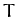. By analogy with Equation (104), we can write such a force as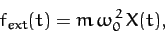(115)

where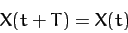(116)

for all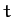.

It is convenient to represent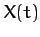as a Fourier series in time, so that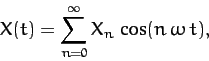(117)

where. By writingin this form, we automatically satisfy the periodicity constraint (116). [Note that by choosing a cosine Fourier series we are limited to even functions in: i.e.,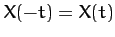. Odd functions incan be represented by sine Fourier series, and mixed functions require a combination of cosine and sine Fourier series.] The constant coefficients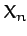are known as Fourier coefficients. But, how do we determine these coefficients for a given functional form,?

Well, it follows from the periodicity of the cosine function that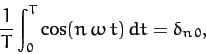(118)

where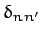is unity if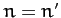, and zero otherwise, and is known as the Kronecker delta function. Thus, integrating Equation (117) infrom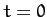to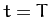, and making use of Equation (118), we obtain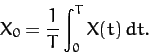(119)

It is also easily demonstrated that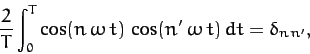(120)

provided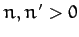. Thus, multiplying Equation (117) by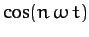, integrating infromto, and making use of Equations (118) and (120), we obtain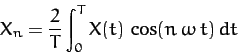(121)

for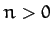. Hence, we have now determined the Fourier coefficients of the general periodic function.

We can incorporate the periodic external force (115) into our perturbed equation of motion by writing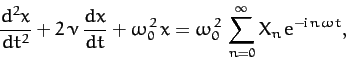(122)

where we are again using the convention that the physical solution corresponds to the real part of the complex solution. Note that the above differential equation is linear. This means that if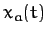and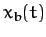represent two independent solutions to this equation then any linear combination ofandis also a solution. We can exploit the linearity of the above equation to write the solution in the form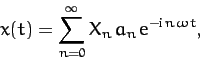(123)

where the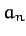are the complex amplitudes of the solutions to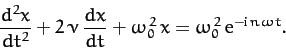(124)

In other words,is obtained by substituting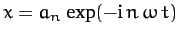into the above equation. Hence, it follows that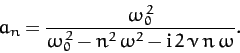(125)

Thus, the physical solution takes the form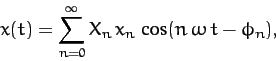(126)

where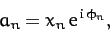(127)

and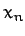and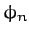are real parameters. It follows from Equation (125) that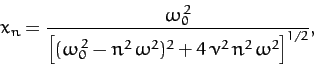(128)

and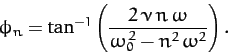(129)

We have now fully determined the response of our dynamical system to a general periodic driving force.

As an example, suppose that the external force periodically delivers a brief kick to the system. For instance, let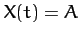for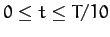and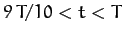, and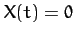otherwise (in the period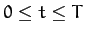). It follows from Equation (119) and (121) that, in this case,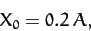(130)

and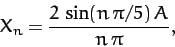(131)

for. Obviously, to obtain an exact solution, we would have to include every Fourier harmonic in Equation (126), which would be impractical. However, we can obtain a fairly accurate approximate solution by truncating the Fourier series (i.e., by neglecting all the terms with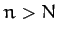, where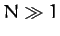).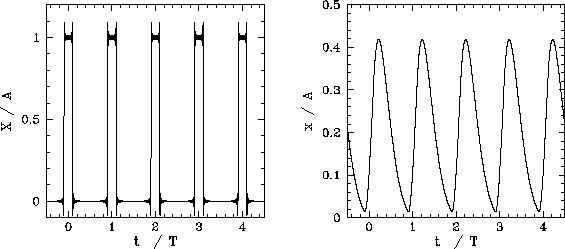Figure 7 shows an example calculation in which the Fourier series is truncated after 100 terms. The parameters used in this calculation are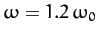and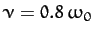. The left panel shows the Fourier reconstruction of the driving force,. The glitches at the rising and falling edges of the pulses are called Gibbs phenomena, and are an inevitable consequence of attempting to represent a discontinuous periodic function as a Fourier series. The right panel shows the Fourier reconstruction of the response,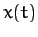, of the dynamical system to the applied force.Next: Transients Up: One-Dimensional Motion Previous: Resonance
Richard Fitzpatrick 2011-03-31